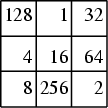## Multiplication Magic SquareA square which is magic under multiplication instead of addition (the operation used to define a conventional Magic Square) is called a multiplication magic square. Unlike (normal) Magic Squares, theentries for anth order multiplicative magic square are not required to be consecutive. The above multiplication magic square has a multiplicative magic constant of 4,096.

Hunter, J. A. H. and Madachy, J. S. Mystic Arrays.'' Ch. 3 in Mathematical Diversions. New York: Dover, pp. 30-31, 1975.